# The MI Procedure

### Monotone Propensity Score Method

The propensity score method is another imputation method available for continuous variables when the data set has a monotone missing pattern.

A propensity score is generally defined as the conditional probability of assignment to a particular treatment given a vector of observed covariates (Rosenbaum and Rubin, 1983). In the propensity score method, for a variable with missing values, a propensity score is generated for each observation to estimate the probability that the observation is missing. The observations are then grouped based on these propensity scores, and an approximate Bayesian bootstrap imputation (Rubin, 1987, p. 124) is applied to each group (Lavori, Dawson, and Shera, 1995).

The propensity score method uses the following steps to impute values for variable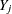with missing values:

1. Creates an indicator variable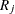with the value 0 for observations with missingand 1 otherwise.

2. Fits a logistic regression model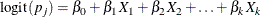where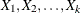are covariates for,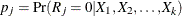,   and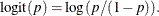3. Creates a propensity score for each observation to estimate the probability that it is missing.

4. Divides the observations into a fixed number of groups (typically assumed to be five) based on these propensity scores.

5. Applies an approximate Bayesian bootstrap imputation to each group. In group k, suppose thatdenotes the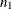observations with nonmissingvalues anddenotes the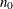observations with missing. The approximate Bayesian bootstrap imputation first drawsobservations randomly with replacement fromto create a new data set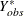. This is a nonparametric analog of drawing parameters from the posterior predictive distribution of the parameters. The process then draws thevalues forrandomly with replacement from.

Steps 1 through 5 are repeated sequentially for each variable with missing values.

The propensity score method was originally designed for a randomized experiment with repeated measures on the response variables. The goal was to impute the missing values on the response variables. The method uses only the covariate information that is associated with whether the imputed variable values are missing; it does not use correlations among variables. It is effective for inferences about the distributions of individual imputed variables, such as a univariate analysis, but it is not appropriate for analyses that involve relationship among variables, such as a regression analysis (Schafer, 1999, p. 11). It can also produce badly biased estimates of regression coefficients when data on predictor variables are missing (Allison, 2000).